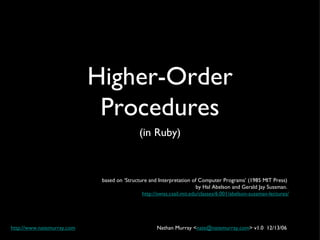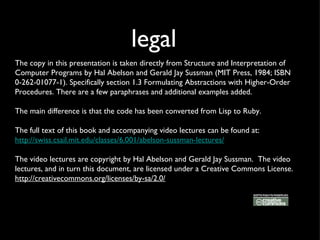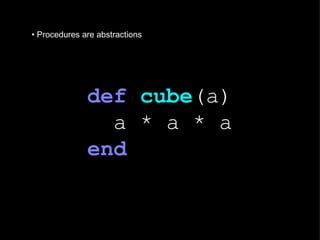Successfully reported this slideshow.

# Higher Order Procedures (in Ruby)

11

Share×
1 of 21
1 of 21

# Higher Order Procedures (in Ruby)

11

Share

The significance of higher-order ... procedures is that they enable us to represent procedural abstractions explicitly as elements in our programming language, so that they can be handled just like other computational elements.

The significance of higher-order ... procedures is that they enable us to represent procedural abstractions explicitly as elements in our programming language, so that they can be handled just like other computational elements.

## More Related Content

### Related Books

Free with a 14 day trial from Scribd

See all

### Related Audiobooks

Free with a 14 day trial from Scribd

See all

### Higher Order Procedures (in Ruby)

1. 1. Higher-Order Procedures <ul><li>(in Ruby) </li></ul>based on ‘Structure and Interpretation of Computer Programs’ (1985 MIT Press) by Hal Abelson and Gerald Jay Sussman. http://swiss.csail.mit.edu/classes/6.001/abelson-sussman-lectures/ Nathan Murray < [email_address] > v1.0 12/13/06 http://www.natemurray.com
2. 2. legal The copy in this presentation is taken directly from Structure and Interpretation of Computer Programs by Hal Abelson and Gerald Jay Sussman (MIT Press, 1984; ISBN 0-262-01077-1). Specifically section 1.3 Formulating Abstractions with Higher-Order Procedures. There are a few paraphrases and additional examples added. The main difference is that the code has been converted from Lisp to Ruby. The full text of this book and accompanying video lectures can be found at: http://swiss.csail.mit.edu/classes/6.001/abelson-sussman-lectures/ The video lectures are copyright by Hal Abelson and Gerald Jay Sussman. The video lectures, and in turn this document, are licensed under a Creative Commons License. http://creativecommons.org/licenses/by-sa/2.0/
3. 3. • Procedures are abstractions def cube (a) a * a * a end
4. 4. ( 3 * 3 * 3 ) (x * x * x) (y * y * y) x 3
5. 5. <ul><li>We need more than numbers for parameters </li></ul><ul><li>Patterns indicate concepts </li></ul><ul><li>Higher-Order Procedures </li></ul><ul><li>Manipulating Procedures = Power </li></ul>
6. 6. some examples <ul><li>Consider the following three procedures. </li></ul>
7. 7. The first computes the sum of the integers from a through b: def sum_integers (a, b) return 0 if a > b a + sum_integers((a + 1 ), b) end sum_integers( 1 , 10 ) #=> 55
8. 8. The second computes the sum of the cubes of the integers in the given range: def sum_cubes (a, b) return 0 if a > b cube(a) + sum_cubes((a + 1 ), b) end sum_cubes( 1 , 3 ) #=> 36
9. 9. The third computes the sum of a sequence of terms in the series: def pi_sum (a, b) return 0 if a > b ( 1.0 / ((a + 2 ) * a)) + (pi_sum((a + 4 ), b)) end pi_sum( 1 , 1000 ) * 8 #=> 3.13959265558978 which converges to π/8 (very slowly)
10. 10. a pattern... def sum_integers (a, b) return 0 if a > b a + sum_integers((a + 1 ), b) end def sum_cubes (a, b) return 0 if a > b cube(a) + sum_cubes((a + 1 ), b) end def pi_sum (a, b) return 0 if a > b ( 1.0 / ((a + 2 ) * a)) + (pi_sum((a + 4 ), b)) end
11. 11. template def <name> (a, b) return 0 if a > b <term>(a) + <name>(< next >(a), b) end
12. 12. summation
13. 13. def <name> (a, b) return 0 if a > b <term>(a) + <name>(< next >(a), b) end def sum (term, a, the_next, b) return 0 if a > b term.call(a) + sum(term, the_next.call(a), the_next, b) end
14. 14. sum cubes def inc (n) n + 1 end def sum_cubes (a, b) cube = self .method( :cube ).to_proc inc = self .method( :inc ).to_proc sum(cube, a, inc, b) end sum_cubes( 1 , 3 ) #=> 36
15. 15. sum integers def identity (x) x end def sum_integers (a, b) id = self .method( :identity ).to_proc inc = self .method( :inc ).to_proc sum(id, a, inc, b) end sum_integers( 1 , 10 ) #=> 55
16. 16. π sum def pi_term (x) ( 1.0 / (x * (x+ 2 ))) end def pi_next (x) (x + 4 ) end def pi_sum (a, b) term = self .method( :pi_term ).to_proc nex = self .method( :pi_next ).to_proc sum(term, a, nex, b) end pi_sum( 1 , 1000 ) * 8 #=> 3.13959265558978 λ
17. 17. λ  def pi_sum (a, b) sum( , a, , b ) end lambda { | x | ( 1.0 / (x * (x+ 2 ))) } lambda { | x | (x + 4 ) }
18. 18. another example def even? (i) i % 2 == 0 end def filter_evens (list) new_list = [] list.each do | element | new_list << element if even?(element) end new_list end filter_evens( [ 1 , 2 , 3 , 4 , 5 , 6 , 7 , 8 ] ) #=> [2, 4, 6, 8]
19. 19. returning procedures def make_filter (predicate) lambda do | list | new_list = [] list.each do | element | new_list << element if predicate.call(element) end new_list end end filter_odds = make_filter( lambda {| i | i % 2 != 0 } ) filter_odds.call(list) #=> [1, 3, 5, 7, 9]
20. 20. returning procedures filter_ths = make_filter( lambda do | i | i.ordinal =~ / th\$ / ? true : false end ) filter_ths.call(list) #=> [4, 5, 6, 7, 8, 9, 10] require ' facet/integer/ordinal ' 10 .ordinal #=> &quot;10th&quot;
21. 21. wrap-up <ul><li>identify abstractions </li></ul><ul><li>abstraction = power </li></ul><ul><li>be appropriate </li></ul>